# Period of a Function

The time interval between two waves is known as a Period whereas a function that repeats its values at regular intervals or periods is known as a Periodic Function. In other words, a periodic function is a function that repeats its values after every particular interval.

The period of the function is this particular interval mentioned above.

A function f will be periodic with period m, so if we have

f (a + m) = f (a), For every m > 0.

It shows that the function f(a) possesses the same values after an interval of “m”. One can say that after every interval of “m” the function f repeats all its values.

For example – The sine function i.e. sin a has a period of 2π because 2π is the smallest number for which sin (a + 2π) = sin a, for all a.

We may also calculate the period using the formula derived from the basic sine and cosine equations. The period for function y = A sin(Bx + C) and y = A cos(Bx + C) is 2π/|B| radians.

The reciprocal of the period of a function = frequency

Frequency is defined as the number of cycles completed in one second. If the period of a function is denoted by P and f be its frequency, then –f =1/ P.

## Fundamental Period of a Function

The fundamental period of a function is the period of the function which are of the form,

f(x+k)=f(x)

f(x+k)=f(x), then k is called the period of the function and the function f is called a periodic function.

Now, let us define the function h(t) on the interval [0, 2] as follows: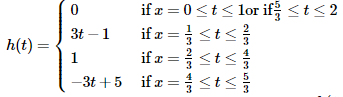If we extend the function h to all of R by the equation,

h(t+2)=h(t)

=> h is periodic with period 2.

The graph of the function is shown below.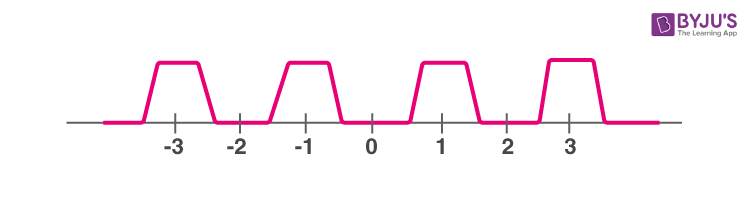## How to Find the Period of a Function?

• If a function repeats over at a constant period we say that is a periodic function.
• It is represented like f(x) = f(x + p), p is the real number and this is the period of the function.
• Period means the time interval between the two occurrences of the wave.

Let’s learn how to fund the period and amplitude of a given trigonometric function, such as sine, cosine, tangent, etc., along with graphs and examples here.

## Period of a Trigonometric Function

The distance between the repetition of any function is called the period of the function. For a trigonometric function, the length of one complete cycle is called a period. For any trigonometry graph function, we can take x = 0 as the starting point.

In general, we have three basic trigonometric functions like sin, cos and tan functions, having -2π, 2π and π periods respectively.

Sine and cosine functions have the forms of a periodic wave: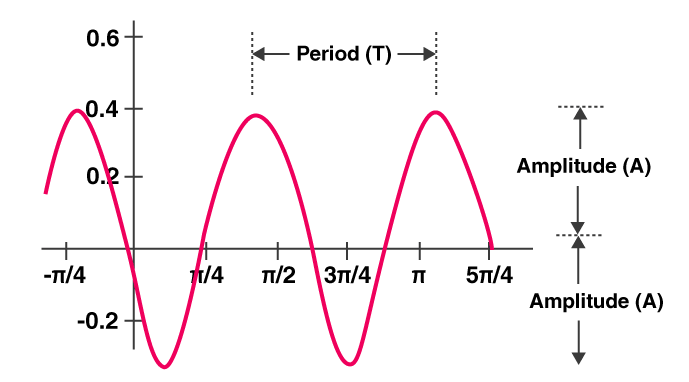• Period: It is represented as “T”. A period is a distance among two repeating points on the graph function.
• Amplitude: It is represented as “A”. It is the distance between the middle point to the highest or lowest point on the graph function.

sin(aθ) = 2πa and cos(aθ) = 2πa

## Period of a Sine Function

If we have a function f(x) = sin (xs), where s > 0, then the graph of the function makes complete cycles between 0 and 2π and each of the function have the period, p = 2π/s

Now, let’s discuss some examples based on sin function:

Let us discuss the graph of y = sin 2x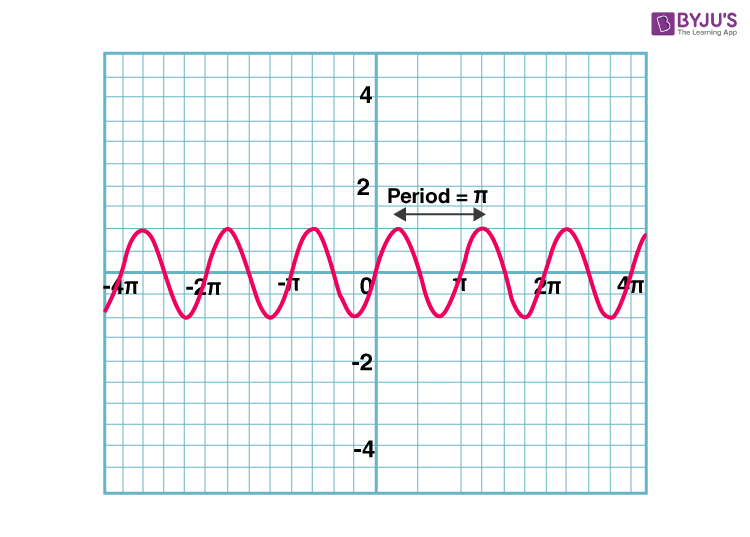Period = π Axis: y = 0 [x-axis ] Amplitude: 1 Maximum value = 1 Minimum value = -1 Domain: { x : x ∈ R } Range = [ -1, 1] –

## Period of a Tangent Function

If we have a function f(a) = tan (as), where s > 0, then the graph of the function makes complete cycles between −π/2, 0 and π/2 and each of the function have the period of p = π/s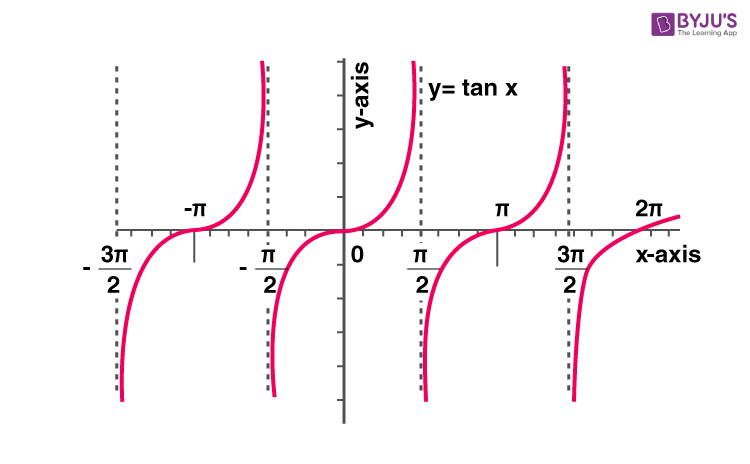### Periodic Functions Examples

Let’s learn some of the examples of periodic functions.

Example 1:

Find the period of the given periodic function f(x) = 9 sin(6x + 5).

Solution:

Given periodic function is f(x) = 9 sin(6x+ 5)

Coefficient of x = B = 6

Period = 2π/ |B|, here period of the periodic function = 2π/ 6 = π/3

Example 2:

What is the period of the following periodic function?

f(a) = 6 cos 5a

Solution:

The given periodic function is f(a) = 6 cos 5a. We have the formula for the period of the function.

Period = 2π/B,

From the given, B = 5

Hence, the period of the given periodic function = 2π/5

Example 3:

Sketch the Graph of y = 4 sin(a/2).

Solution:

Given,

y = 4 sin(a/2)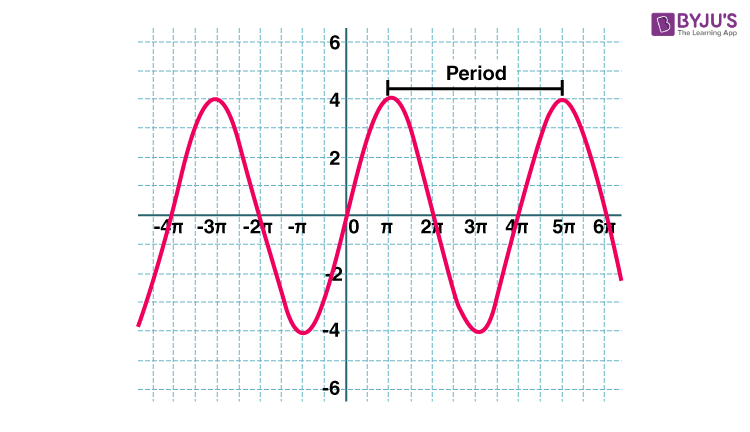From the above graph, we can write the following:

• Period = 4π
• Axis: y = 0 [x-axis ]
• Amplitude: 4
• Maximum value = 4
• Minimum value = -4
• Domain: { x : x ∈ R }
• Range = [ -4, 4]

### Practice Problems

1. Find the period of the function f(x) = 3 cos 2x.
2. Find the period and amplitude of the function y = 2 sin(6x – π) + 4.
3. What is the period of the function y = 5 sin x – 7 sin 8x?

Keep visiting BYJU’S – The Learning App and download the app from the Google Play store and explore more videos to learn with ease.

Test your Knowledge on Period of a Function